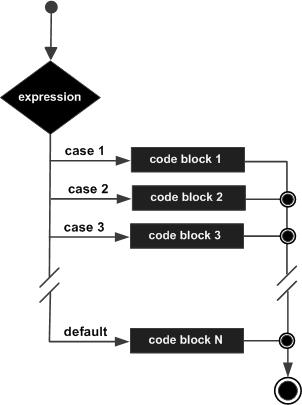# Powershell - Switch Statement

A switch statement allows a variable to be tested for equality against a list of values. Each value is called a case, and the variable being switched on is checked for each case.

## Syntax

The syntax of enhanced for loop is −

```switch(<test-value>) {
<condition> {<action>}
break; // optional
<condition> {<action>}
break; // optional
<condition> {<action>}
break; // optional
}
```

The following rules apply to a switch statement −

• The variable used in a switch statement can only be any object or an array of objects.

• You can have any number of case statements within a switch. Each case is followed by optional action to be performed.

• The value for a case must be the same data type as the variable in the switch and it must be a constant or a literal.

• When the variable being switched on is equal to a case, the statements following that case will execute until a break statement is reached.

• When a break statement is reached, the switch terminates, and the flow of control jumps to the next line following the switch statement.

• Not every case needs to contain a break. If no break appears, the flow of control will fall through to subsequent cases until a break is reached.

## Flow Diagram## Example 1

Example of Switch statment without break statment.

```switch(3){
1 {"One"}
2 {"Two"}
3 {"Three"}
4 {"Four"}
3 {"Three Again"}
}
```

This will produce the following result −

```Three
Three Again
```

## Example 2

Example of Switch statment with break statment.

```switch(3){
1 {"One"}
2 {"Two"}
3 {"Three"; break }
4 {"Four"}
3 {"Three Again"}
}
```

This will produce the following result −

```Three
```

## Example 3

Example of Switch statment with array as input.

```switch(4,2){
1 {"One"}
2 {"Two"}
3 {"Three"; break }
4 {"Four"}
3 {"Three Again"}
}
```

This will produce the following result −

## Output

```Four
Two
```
powershell_conditions.htm